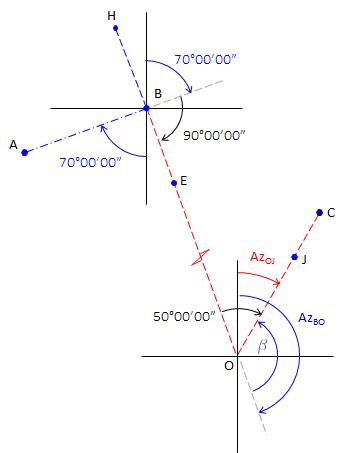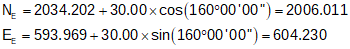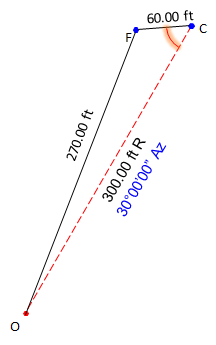## 2. Intersecting Curves

### a. Street Lines

 Coordinates of point A are 2000.00' N and 500.00' E. Using the curve geometry shown, compute coordinates of the labeled points.Because sine and cosine of Azimuths will always return the correct mathematical sign in coordinate computations, they will be used instead of Bearings. Starting at point A, compute azimuths to the side points and all the way to point CSide points G and D at A.Side points H and E at B; Continue to center of arc then cul-de-sac center.Compute sideline points D and G coordinates from point A:Compute point B from point A:Then the sideline points H and E from B:Starting at point B, compute points O, J, and C:Point F cannot be directly computed from a known point. Because its position is defined by the intersection of the southerly street sideline and the cul-de-sac, it is connected by radii to points O and C. Its position is computed from a distance-distance intersectionWe have the length and direction of the baseline OC. We also have lengths of the other two sides of the intersection triangle: dCF = 60.00' and dOF = 300.00'-30.00' = 270.00' Because a distance-distance intersection results in two possible point locations, we must take care to solve the correct triangle.Use Law of Cosines to solve angle at C:Then the direction from C to F:And finally the coordinates from point C:Similarly, point I is at the intersection of the northerly street sideline and the cul-de-sac. Intersection traingle sides are 300.00', 60.00' and 330.00'. As before, there are two possible intersection locations. After determining correct triangle to solve:Use Law of Cosines to solve angle at C:Then the direction from C to I:And finally the coordinates from point C:Coordinates Summary: